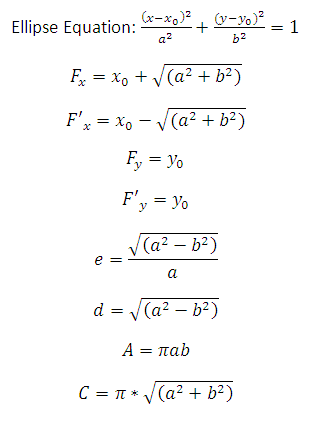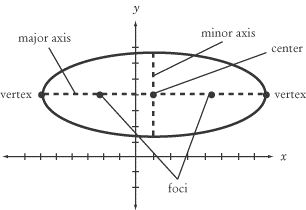# Ellipse Calculator

This CalcTown calculator calculates the focii, eccentricity, area and circumference of an ellipse.

#### Result

m
m
m
m
m
m2
mWhere,

x0, y0 = center of the ellipse

a = semi-major axis length of the ellipse

b = semi-minor axis of the ellipse

Fx, Fy= 1st focus of the ellipse

F'x, F'= 2nd focus of the ellipse

e = eccentricity of the ellipse

d = distance from center to any one of the focii of the ellipse.

A = area of the ellipse.

C = circumference of the ellipse.